Like   Tweet   Pin   +1   in

On Wednesday November 28, 7.30-11pm, we invite you to RU's 9th BENEFIT PARTY held at World of McIntosh Townhouse (WoM) for our annual fund raiser with installations by RU artists, performances by Illustrious Blacks and a holiday choir, our (naughty) Santa, delicious foods by chef Jazzie Einalhori, a gong concert by David Shemesh and more. Purchase your ticket here.
RU's signature raffle draw will take place that evening. The winning artist will get a fully funded residency co-hosted by REMONT Gallery in Belgrade, and Naked Head Residency that is set in the beautiful Serbian countryside close to the capital. Get raffle tickets as an artist or artist supporter !

INCOMING RU RESIDENTS
This month, we are proud to welcome two international artists:
- Mateusz Sarzyński (Poland), winner of the 2018 Hestia Artistic Journey Award whose work you can discover here;
- Winner Jumalon (Philippines) who is an Asian Cultural Council fellow grantee, and considered one of the most compelling Filipino artists of his generation.

NOVEMBER PROGRAMS AND EXHIBITIONS

▪ Nov 1, 1pm Meet Over Lunch: The Good Death Roundtable with Heide Hatry (Icons in Ash), moderated by Bethany Tabor and co-organized with Let's Reimagine End of Life;
▪ Nov 2, 6.30pm RU Exhibition: Portal at a divided fountain, an immersive visual and sound installation by the RU artist Liza Grobler and percussionist Heinrich Kruse, both from South Africa;
▪ Nov 15, 6.30pm RU Talk: RU artists and YVAA fellows Remijon Pronja and Lőrinc Borsos discuss their practice with Shimrit Lee, RU curator in residence;
▪ Nov 16, 6pm RU Exhibition: mono no aware, a group exhibition of new work by RU artists curated by Eric Sutphin. With Joanna Borkowska, Emilie Regnier, Oneika Russel, and Meggy Rustamova; We are truly grateful to Realty Collective for generously hosting this exhibition;
▪ Nov 17, 12-6pm Open Studio: art duo from Hungary Lőrinc Borsos invite RU audiences to their studio at 42 Hawthorne St, Lefferts Gardens;
▪ Nov 20, 6.30pm RU Talk: Marcela Guerrero, Assistant Curator at the Whitney Museum of American Art in dialogue with the Cuban artist Glexis Novoa.
 ▪ Nov 1, 1pm Meet Over Lunch: The Good Death Roundtable with Heide Hatry (Icons in Ash), moderated by Bethany Tabor and co-organized with Let's Reimagine End of Life;
 ▪ Nov 2, 6.30pm RU Exhibition: Portal at a divided fountain, an immersive visual and sound installation by the RU artist Liza Grobler and percussionist Heinrich Kruse, both from South Africa;
 ▪ Nov 15, 6.30pm RU Talk: RU artists and YVAA fellows Remijon Pronja and Lőrinc Borsos discuss their practice with Shimrit Lee, RU curator in residence;
 ▪ Nov 16, 6pm RU Exhibition: mono no aware, a group exhibition of new work by RU artists curated by Eric Sutphin. With Joanna Borkowska, Emilie Regnier, Oneika Russel, and Meggy Rustamova; We are truly grateful to Realty Collective for generously hosting this exhibition;
 ▪ Nov 17, 12-6pm Open Studio: art duo from Hungary Lőrinc Borsos invite RU audiences to their studio at 42 Hawthorne St, Lefferts Gardens;
 ▪ Nov 20, 6.30pm RU Talk: Marcela Guerrero, Assistant Curator at the Whitney Museum of American Art in dialogue with the Cuban artist Glexis Novoa.

OTHER RU RELATED NEWS
-RU is proud to launch its collaboration with the Realty Collective space located in Red Hook, which will host a series of RU programs starting this month with the mono no aware exhibition. Realty Collective envisions an experience of Brooklyn real estate where people feel in charge of their own process, rather than overwhelmed by it.

-On Nov 8 and 9 at NYU's Kevorkian Center, RU curator Shimrit Lee is organizing the panel Seeing Landscape in Israel/Palestine. Speakers include the RU Israeli Druze artist Fatma Shanan who will speak about “The Rug as Heterotopic Zone” on Nov 9 in the morning.

-Rashwan Abdelbaki, the 2018 RU/Safe Haven resident artist, presents his paintings in a solo show in Washington at SYRA Arts: One Eye Opened, One Eye Closed, highlighting the context of the Syrian war and underlying social crises experienced by citizens of the Middle East. On view Nov 15-Dec 15.

-New York based RU alumni Farideh Sakhaeifar and Onyedika Chuke are among fifty-two New York­–based emerging artists commissioned to create new work for Shed's (NY) inaugural Open Call program.

-This month's featured articles on RU Dialogues present director Tuula Paalimäki who speaks about Ars Longa house and its studio building project for senior artists at the Finnish Artists' Studio Foundation in Helsinki. We then jump across the Mediterranean with Dr Zoe Bray's research into the potential development of urban artist-run residencies in East Jerusalem and their function in an area characterized by nationalist, ethnic and religious clashes.

 table div table+table+table div table{width:100%;padding:0}table div table+table+table div table img{width:96.23%;padding:0;float:none}table div table+table+table div table td{width:100%;padding:0 1.88% 18px}/* styles *//* styles */ If you cant make it to the benefit, we invite you to make a donation...
 table div table+table+table+table+table+table div table{width:100%;padding:0}table div table+table+table+table+table+table div table img{width:96.23%;padding:0;float:none}table div table+table+table+table+table+table div table td{width:100%;padding:0 1.88% 18px}/* styles */## Meet Over Lunch: The Good Death Roundtable with Heide Hatry (Icons in Ash)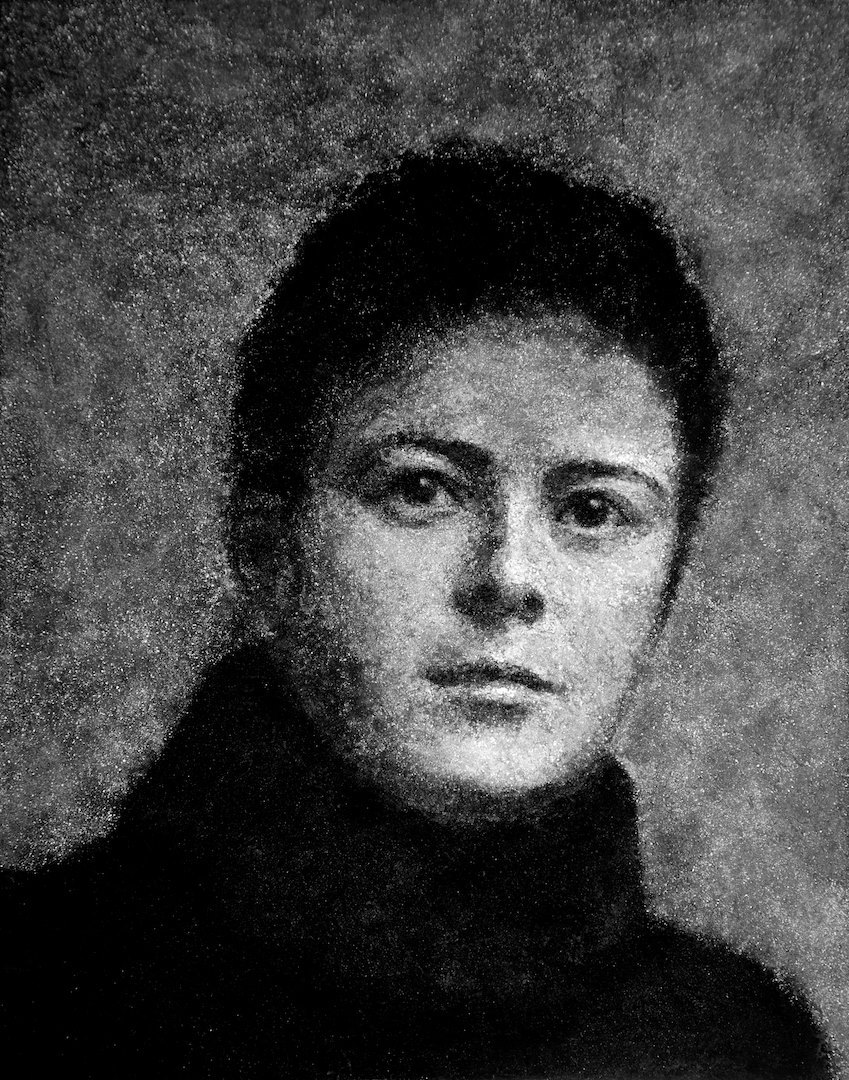Heide Hatry. Lena Sereda, 2016. Mosaic (loose cremated ash particles from Lena Sereda, pulverized birch coal, and white marble dust on beeswax) 14 x 11 in.

Nov 1, 1pm at RU

Join Bethany Tabor and Icons in Ash artist Heide Hatry at this special Good Death Roundtable session to consider and discuss the intersection of artistic practice and alternative modes of memorialization. A program co-organized with Let's Reimagine End of Life.

Reimagine End of Life is a week of exploring big questions about life and death through art, creativity, and conversation.

 table div table+table+table+table+table+table+table+table+table+table div table{width:100%;padding:0}table div table+table+table+table+table+table+table+table+table+table div table img{width:96.23%;padding:0;float:none}table div table+table+table+table+table+table+table+table+table+table div table td{width:100%;padding:0 1.88% 18px}/* styles */## RU Exhibition: Portal at a divided fountain, Liza Grobler and Heinrich Kruse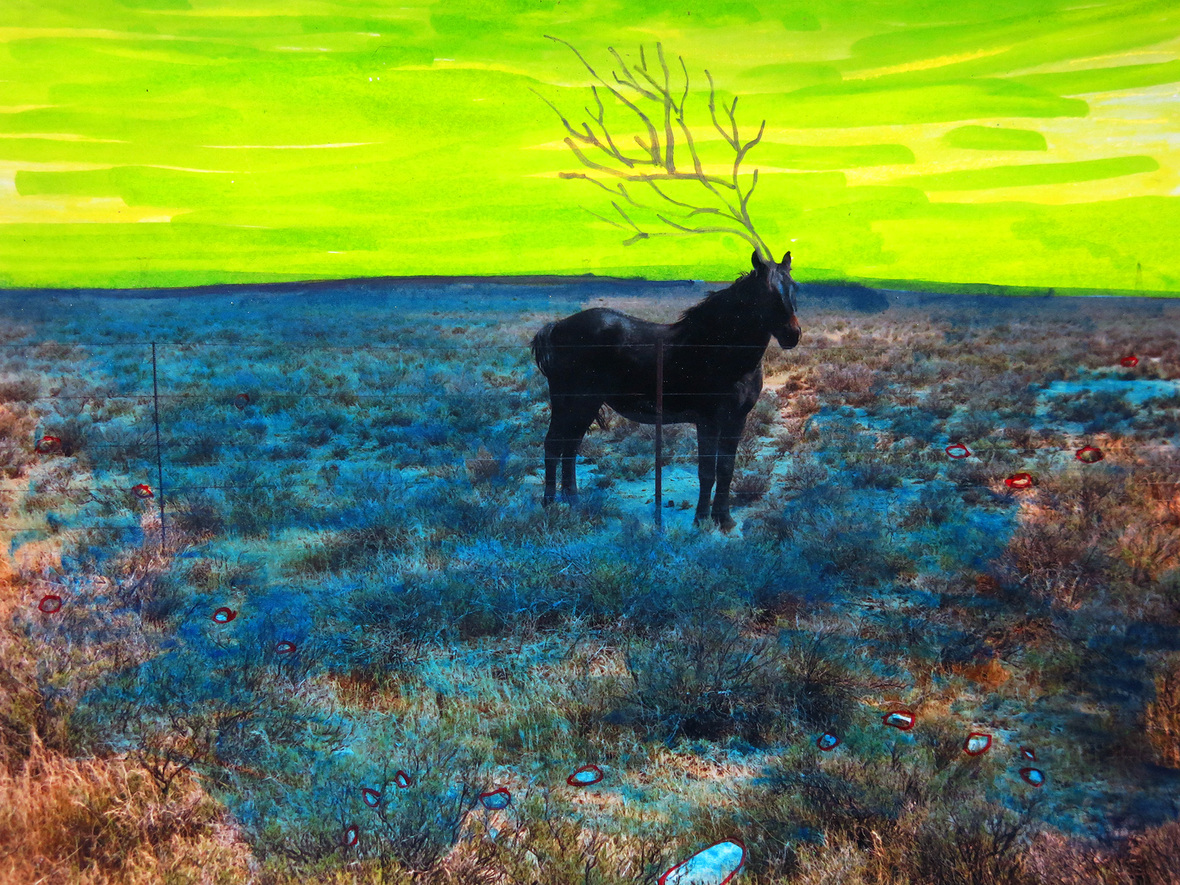Nov 2 at 6.30pm at RU

Portal at a divided fountain, by South African artist Liza Grobler, and percussionist Heinrich Kruse is a multi-sensory tale from the Southern tip of Africa. Sixty drawings in sixty minutes will usurp the borders of time set against percussive rhythms an immersive visual narration and installation.

 table div table+table+table+table+table+table+table+table+table+table+table+table+table div table{width:100%;padding:0}table div table+table+table+table+table+table+table+table+table+table+table+table+table div table img{width:96.23%;padding:0;float:none}table div table+table+table+table+table+table+table+table+table+table+table+table+table div table td{width:100%;padding:0 1.88% 18px}/* styles */## RU Talk: Remijon Pronja and Lőrinc Borsos in conversation with Shimrit Lee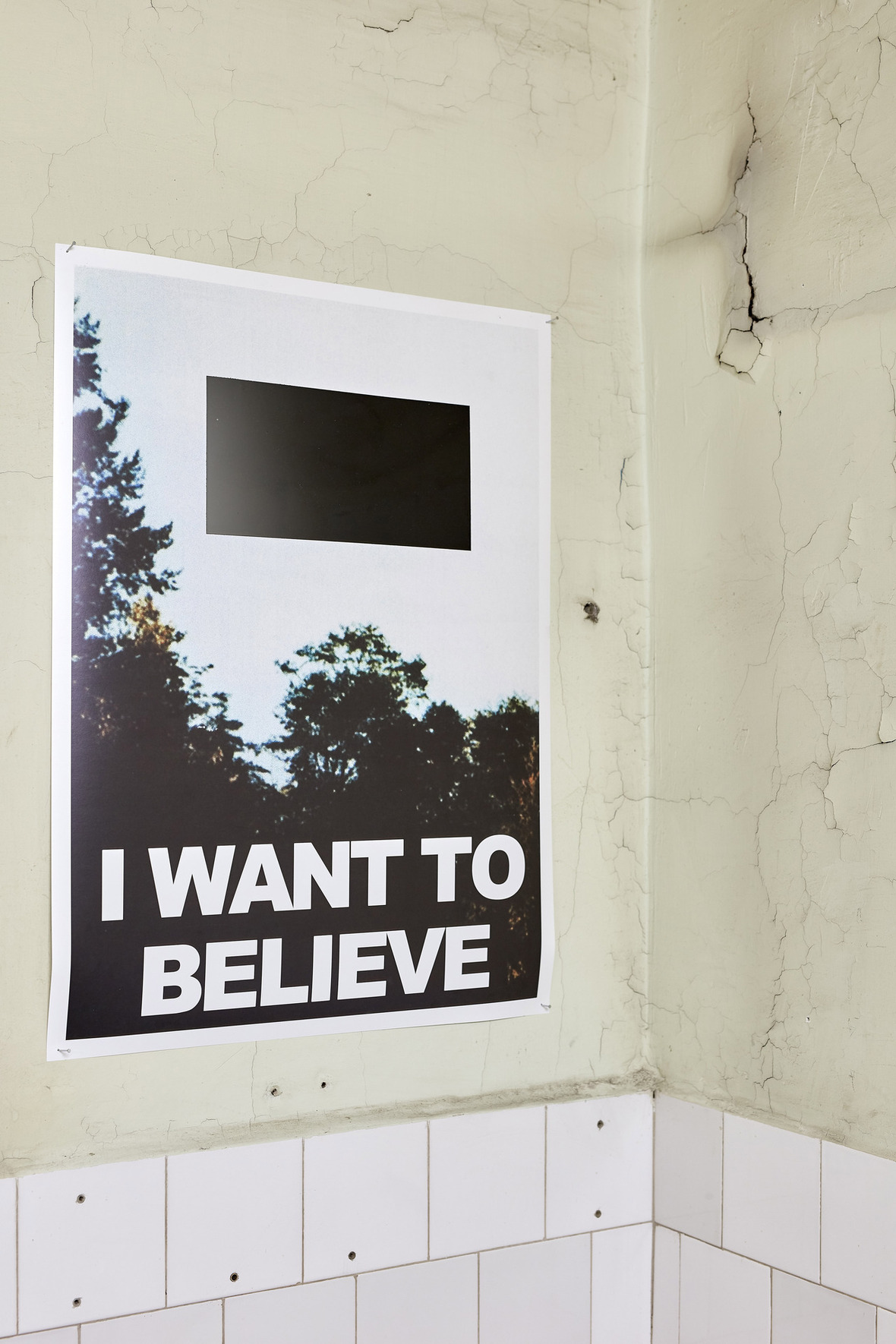Lőrinc Borsos: 'I really want to', 2018 (print on paper, enamel, A/2). Photo: Dávid Biró

Nov 15, 6.30pm at RU

The practice of “cultural editing”—the modification of certain national, religious, or personal symbols to stimulate new modalities of knowledge—is prevalent in the work of RU artists and YVAA fellows Remijon Pronja from Albania and the Hungarian duo Lőrinc Borsos. As the moderator of this discussion, the New York based curator Shimrit Lee looks at these respective artistic practices in relation to history, iconography, censorship and European identity.

In addition, Lőrinc Borsos invite RU audiences to visit their studio at 42 Hawthorne St, Lefferts Gardens for an Open Studio event.

 table div table+table+table+table+table+table+table+table+table+table+table+table+table+table+table+table div table{width:100%;padding:0}table div table+table+table+table+table+table+table+table+table+table+table+table+table+table+table+table div table img{width:96.23%;padding:0;float:none}table div table+table+table+table+table+table+table+table+table+table+table+table+table+table+table+table div table td{width:100%;padding:0 1.88% 18px}/* styles */## RU Exhibition: mono no aware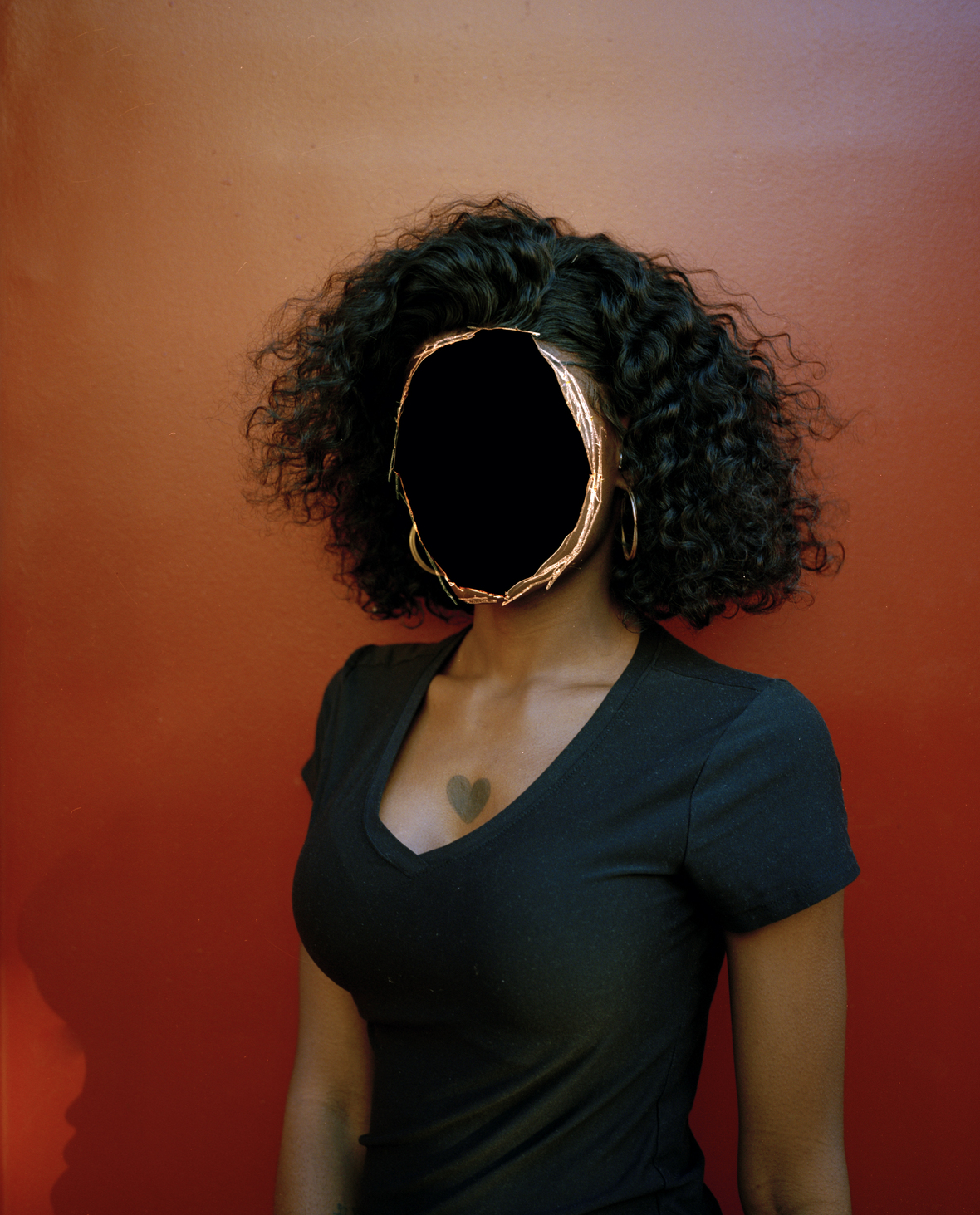Émilie Régnier: Anonyme, 2018.

Nov 16, 6pm at Realty Collective in Red Hook

In considering mono no aware, we can think of William James’s dictum “My experience is what I agree to attend to. Only those items which I notice shape my mind as guide along the way...” Join us for the opening of this exhibition curated by Eric Sutphin featuring new work by RU artists Joanna Borkowska, Emilie Regnier, Oneika Russel, and Meggy Rustamova.

 table div table+table+table+table+table+table+table+table+table+table+table+table+table+table+table+table+table+table+table div table{width:100%;padding:0}table div table+table+table+table+table+table+table+table+table+table+table+table+table+table+table+table+table+table+table div table img{width:96.23%;padding:0;float:none}table div table+table+table+table+table+table+table+table+table+table+table+table+table+table+table+table+table+table+table div table td{width:100%;padding:0 1.88% 18px}/* styles */## RU Talk: Glexis Novoa in dialogue with Marcela Guerrero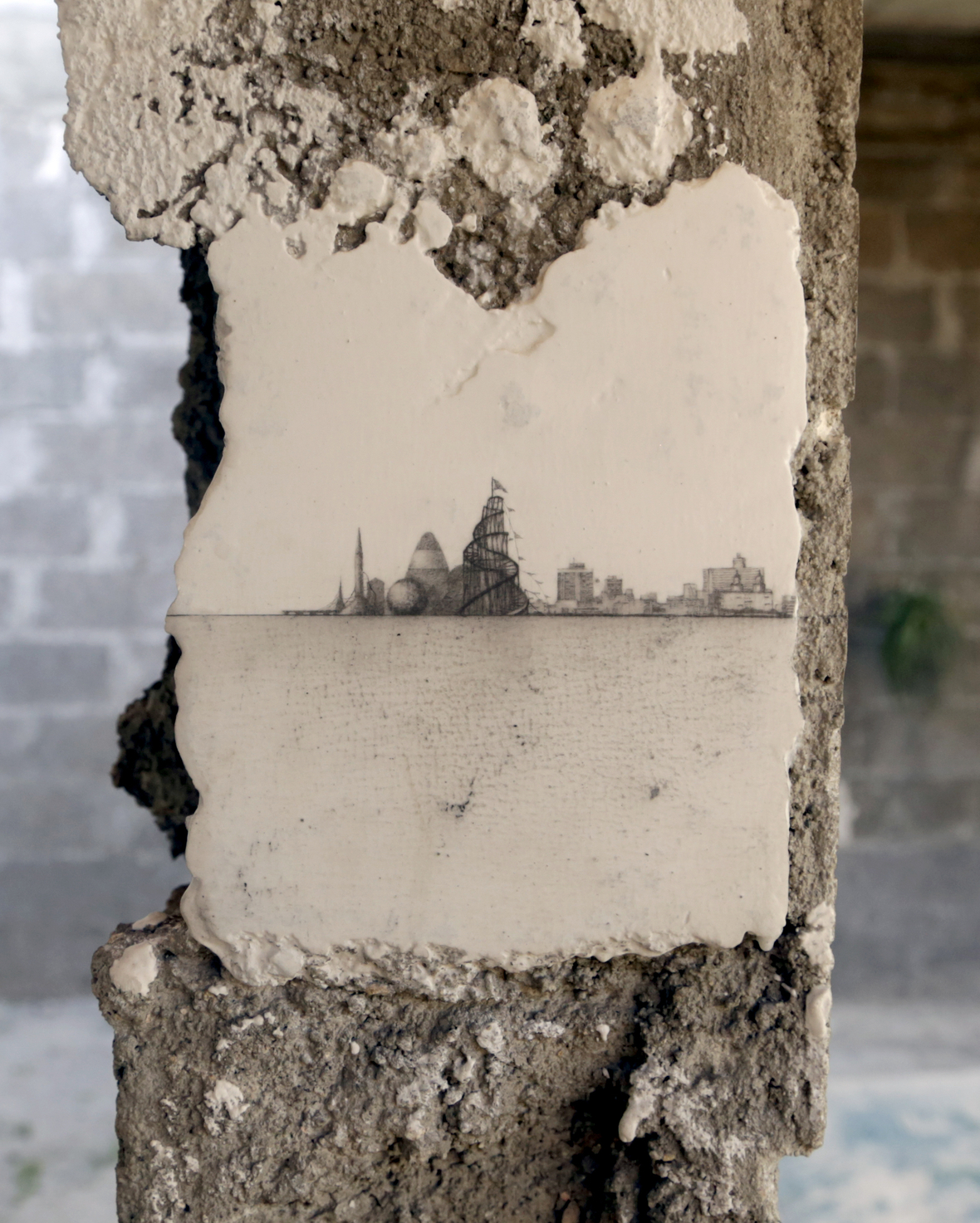Glexis Novoa: Emptiness, 2015. site-specific graphite drawing at an abandoned building inhabited by homeless; XII Havana Biennial, Havana

Nov 20, 6.30pm at RU

In dialogue with Marcela Guerrero, assistant curator at the Whitney Museum, the RU Miami/Havana based artist Glexis Novoa will explain how his use of multimedia and drawing on marble act as an instrument of social and political criticism. This will be an opportunity to see new works realized during Novoa's residency at RU.

 table div table+table+table+table+table+table+table+table+table+table+table+table+table+table+table+table+table+table+table+table+table+table div table{width:100%;padding:0}table div table+table+table+table+table+table+table+table+table+table+table+table+table+table+table+table+table+table+table+table+table+table div table img{width:96.23%;padding:0;float:none}table div table+table+table+table+table+table+table+table+table+table+table+table+table+table+table+table+table+table+table+table+table+table div table td{width:100%;padding:0 1.88% 18px}/* styles */## RU Alumni News:

 /* styles */ We invite you to visit Dense Lightness, an exhibition of works by Ivan Forde, curated by RU alum Ana Harsanyi, at Baxter St at CCNY (126 Baxter St). The opening will be on Thursday, November 8, 6-8pm, and the exhibition is on view until December 15.
 table div table+table+table+table+table+table+table+table+table+table+table+table+table+table+table+table+table+table+table+table+table+table+table+table+table div table{width:100%;padding:0}table div table+table+table+table+table+table+table+table+table+table+table+table+table+table+table+table+table+table+table+table+table+table+table+table+table div table table{padding:0;float:left!important;width:26.226%!important}table div table+table+table+table+table+table+table+table+table+table+table+table+table+table+table+table+table+table+table+table+table+table+table+table+table div table table+table+table+table td,table div table+table+table+table+table+table+table+table+table+table+table+table+table+table+table+table+table+table+table+table+table+table+table+table+table div table td{padding-left:0;padding-right:0}table div table+table+table+table+table+table+table+table+table+table+table+table+table+table+table+table+table+table+table+table+table+table+table+table+table div table table td,table div table+table+table+table+table+table+table+table+table+table+table+table+table+table+table+table+table+table+table+table+table+table+table+table+table div table table+table td,table div table+table+table+table+table+table+table+table+table+table+table+table+table+table+table+table+table+table+table+table+table+table+table+table+table div table table+table+table td{padding-left:0;padding-right:20px}table div table+table+table+table+table+table+table+table+table+table+table+table+table+table+table+table+table+table+table+table+table+table+table+table+table div table table+table{float:left!important;width:25.66%!important}table div table+table+table+table+table+table+table+table+table+table+table+table+table+table+table+table+table+table+table+table+table+table+table+table+table div table table+table+table{float:left!important;width:26.037%!important}table div table+table+table+table+table+table+table+table+table+table+table+table+table+table+table+table+table+table+table+table+table+table+table+table+table div table table+table+table+table{float:left!important;width:22.076999999999998%!important}/* styles */
 table div table+table+table+table+table+table+table+table+table+table+table+table+table+table+table+table+table+table+table+table+table+table+table+table+table+table div table{width:100%;padding:0}table div table+table+table+table+table+table+table+table+table+table+table+table+table+table+table+table+table+table+table+table+table+table+table+table+table+table div table table{padding:0;float:left!important;width:26.226%!important}table div table+table+table+table+table+table+table+table+table+table+table+table+table+table+table+table+table+table+table+table+table+table+table+table+table+table div table table+table+table+table td,table div table+table+table+table+table+table+table+table+table+table+table+table+table+table+table+table+table+table+table+table+table+table+table+table+table+table div table td{padding-left:0;padding-right:0}table div table+table+table+table+table+table+table+table+table+table+table+table+table+table+table+table+table+table+table+table+table+table+table+table+table+table div table table td,table div table+table+table+table+table+table+table+table+table+table+table+table+table+table+table+table+table+table+table+table+table+table+table+table+table+table div table table+table td,table div table+table+table+table+table+table+table+table+table+table+table+table+table+table+table+table+table+table+table+table+table+table+table+table+table+table div table table+table+table td{padding-left:0;padding-right:20px}table div table+table+table+table+table+table+table+table+table+table+table+table+table+table+table+table+table+table+table+table+table+table+table+table+table+table div table table+table,table div table+table+table+table+table+table+table+table+table+table+table+table+table+table+table+table+table+table+table+table+table+table+table+table+table+table div table table+table+table{float:left!important;width:25.849%!important}table div table+table+table+table+table+table+table+table+table+table+table+table+table+table+table+table+table+table+table+table+table+table+table+table+table+table div table table+table+table+table{float:left!important;width:22.075999999999993%!important}/* styles */
 table div table+table+table+table+table+table+table+table+table+table+table+table+table+table+table+table+table+table+table+table+table+table+table+table+table+table+table div table{width:100%;padding:0}table div table+table+table+table+table+table+table+table+table+table+table+table+table+table+table+table+table+table+table+table+table+table+table+table+table+table+table div table table{padding:0;float:left!important;width:26.037%!important}table div table+table+table+table+table+table+table+table+table+table+table+table+table+table+table+table+table+table+table+table+table+table+table+table+table+table+table div table table+table+table+table td,table div table+table+table+table+table+table+table+table+table+table+table+table+table+table+table+table+table+table+table+table+table+table+table+table+table+table+table div table td{padding-left:0;padding-right:0}table div table+table+table+table+table+table+table+table+table+table+table+table+table+table+table+table+table+table+table+table+table+table+table+table+table+table+table div table table+table+table{float:left!important;width:26.037%!important}table div table+table+table+table+table+table+table+table+table+table+table+table+table+table+table+table+table+table+table+table+table+table+table+table+table+table+table div table table td,table div table+table+table+table+table+table+table+table+table+table+table+table+table+table+table+table+table+table+table+table+table+table+table+table+table+table+table div table table+table td,table div table+table+table+table+table+table+table+table+table+table+table+table+table+table+table+table+table+table+table+table+table+table+table+table+table+table+table div table table+table+table td{padding-left:0;padding-right:20px}table div table+table+table+table+table+table+table+table+table+table+table+table+table+table+table+table+table+table+table+table+table+table+table+table+table+table+table div table table+table{float:left!important;width:26.226%!important}table div table+table+table+table+table+table+table+table+table+table+table+table+table+table+table+table+table+table+table+table+table+table+table+table+table+table+table div table table+table+table+table{float:left!important;width:21.700000000000003%!important}/* styles */
 table div table+table+table+table+table+table+table+table+table+table+table+table+table+table+table+table+table+table+table+table+table+table+table+table+table+table+table+table div table{width:100%;padding:0}table div table+table+table+table+table+table+table+table+table+table+table+table+table+table+table+table+table+table+table+table+table+table+table+table+table+table+table+table div table table{padding:0;float:left!important;width:26.011%!important}table div table+table+table+table+table+table+table+table+table+table+table+table+table+table+table+table+table+table+table+table+table+table+table+table+table+table+table+table div table td{padding-left:5px;padding-right:5px}table div table+table+table+table+table+table+table+table+table+table+table+table+table+table+table+table+table+table+table+table+table+table+table+table+table+table+table+table div table table+table{float:left!important;width:26.011%!important}table div table+table+table+table+table+table+table+table+table+table+table+table+table+table+table+table+table+table+table+table+table+table+table+table+table+table+table+table div table table td,table div table+table+table+table+table+table+table+table+table+table+table+table+table+table+table+table+table+table+table+table+table+table+table+table+table+table+table+table div table table+table td,table div table+table+table+table+table+table+table+table+table+table+table+table+table+table+table+table+table+table+table+table+table+table+table+table+table+table+table+table div table table+table+table td{padding-left:0;padding-right:20px}table div table+table+table+table+table+table+table+table+table+table+table+table+table+table+table+table+table+table+table+table+table+table+table+table+table+table+table+table div table table+table+table{float:left!important;width:25.818%!important}table div table+table+table+table+table+table+table+table+table+table+table+table+table+table+table+table+table+table+table+table+table+table+table+table+table+table+table+table div table table+table+table+table{float:left!important;width:22.159999999999997%!important}table div table+table+table+table+table+table+table+table+table+table+table+table+table+table+table+table+table+table+table+table+table+table+table+table+table+table+table+table div table table+table+table+table td{padding-left:0;padding-right:0}/* styles */
 table div table+table+table+table+table+table+table+table+table+table+table+table+table+table+table+table+table+table+table+table+table+table+table+table+table+table+table+table+table div table{width:100%;padding:0}table div table+table+table+table+table+table+table+table+table+table+table+table+table+table+table+table+table+table+table+table+table+table+table+table+table+table+table+table+table div table img{width:96.23%;padding:0;float:none}table div table+table+table+table+table+table+table+table+table+table+table+table+table+table+table+table+table+table+table+table+table+table+table+table+table+table+table+table+table div table td{width:100%;padding:0 1.88% 18px}/* styles */## Join RU Membership Cycle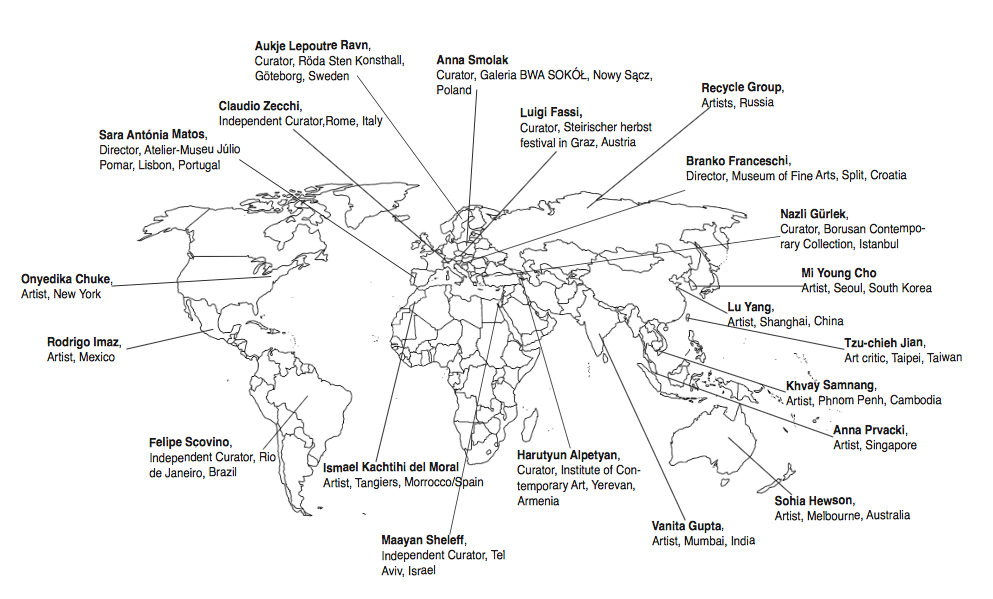By becoming RU member you provide critical support for our residency program and year-round free public programs! The membership includes studio visits with RU residents, private exhibition previews, guided tours to RU art commissions, and access to RU’s global network.

 table div table+table+table+table+table+table+table+table+table+table+table+table+table+table+table+table+table+table+table+table+table+table+table+table+table+table+table+table+table+table+table+table div table{width:100%;padding:0}table div table+table+table+table+table+table+table+table+table+table+table+table+table+table+table+table+table+table+table+table+table+table+table+table+table+table+table+table+table+table+table+table div table img{width:96.23%;padding:0;float:none}table div table+table+table+table+table+table+table+table+table+table+table+table+table+table+table+table+table+table+table+table+table+table+table+table+table+table+table+table+table+table+table+table div table td{width:100%;padding:0 1.88% 18px}/* styles */